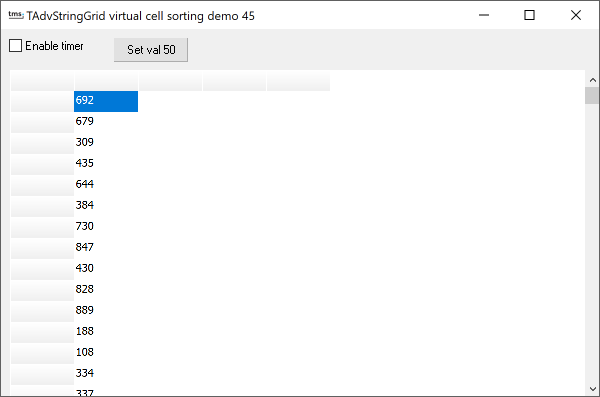Example 45 : virtual cell sortingThis example combines the techniques of using virtual cell as discussed in example 41 with sort independent cell access shown in example 40.

In this sample, the values shown in the grid column 1 are not stored in the grid cells itself but in an array of integer values declared as:

values : array[0..100] of
integer;

The normal way for using this array of values as virtual cell content for column 1 would be:

ARow: Integer; var Value: String);
begin
if (ACol = 1) and (ARow > 0) then
Value := InttoStr(Values[ARow]);
end;

Note that with this approach, the grid would always display values in visual row 1, values in visual row 2 etc ... We do want though that the grid display can be sorted (without resorting to sorting the values array as well) This can be achieved by using the sort-independent row access as discussed in example 40 and thus becomes:

ARow: Integer; var Value: String);
begin
if (ACol = 1) and (ARow > 0) then